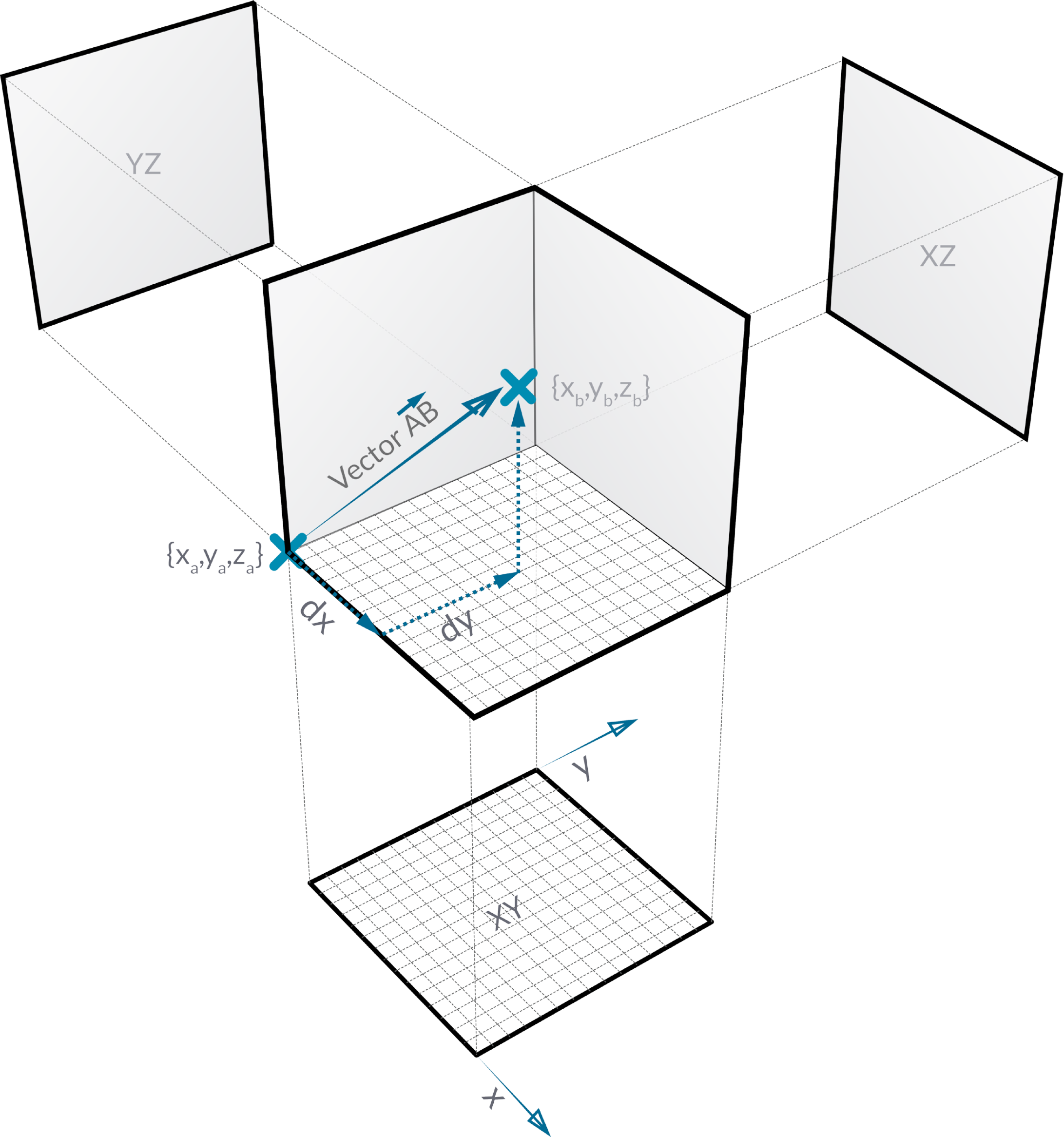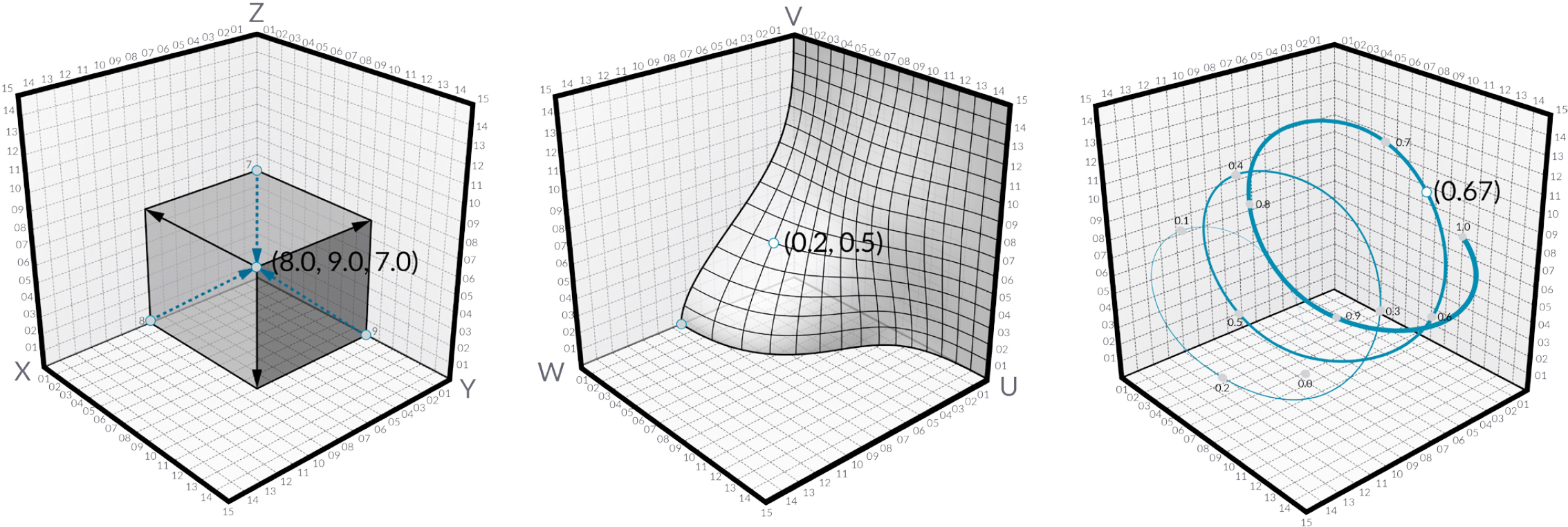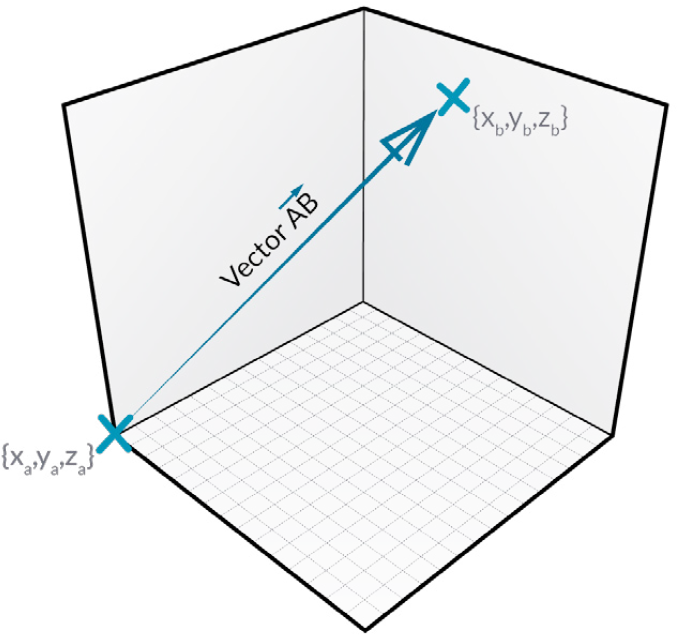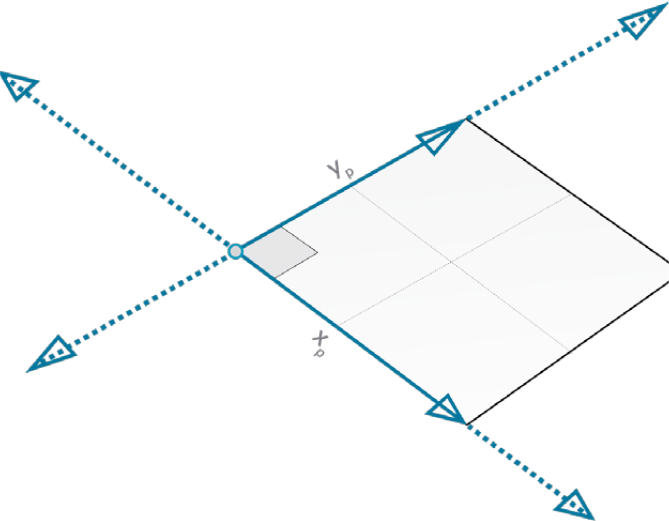### 1.3.1. Points, Planes & Vectors

##### Everything begins with points. A point is nothing more than one or more values called coordinates. The number of coordinate values corresponds with the number of dimensions of the space in which it resides. Points, planes, and vectors are the base for creating and transforming geometry in Grasshopper.#### 1.3.1.1 POINTS

Points in 3D space have three coordinates, usually referred to as [x,y,z]. Points in 2D space have only two coordinates which are either called [x,y] or [u,v] depending on what kind of two dimensional space we’re talking about. 2D parameter space is bound to a finite surface. It is still continuous, I.e. hypothetically there are an infinite amount of points on the surface, but the maximum distance between any of these points is very much limited. 2D parameter coordinates are only valid if they do not exceed a certain range. In the example drawing, the range has been set between 0.0 and 1.0 for both [u] and [v] directions, but it could be any finite domain. A point with coordinates [1.5, 0.6] would be somewhere outside the surface and thus invalid.

Since the surface which defines this particular parameter space resides in regular 3D world space, we can always translate a parametric coordinate into a 3D world coordinate. The point [0.2, 0.5] on the surface for example is the same as point [1.8, 2.0, 4.1] in world coordinates. Once we transform or deform the surface, the 3D coordinates which correspond with [0.2, 0.5] will change.If this is a hard concept to grasp, it might help to think of yourself and your position in space. We tend to use local coordinate systems to describe our whereabouts; “I’m sitting in the third seat on the seventh row in the movie theatre”, “I’m in the back seat”. If the car you’re in is on the road, your position in global coordinates is changing all the time, even though you remain in the same back seat ‘coordinate’.

#### 1.3.1.2. VECTORS

A vector is a geometric quantity describing Direction and Magnitude. Vectors are abstract; ie. they represent a quantity, not a geometrical element.

Vectors are indistinguishable from points. That is, they are both lists of three numbers so there’s absolutely no way of telling whether a certain list represents a point or a vector. There is a practical difference though; points are absolute, vectors are relative. When we treat a list of three doubles as a point it represents a certain coordinate in space, when we treat it as a vector it represents a certain direction. A vector is an arrow in space which always starts at the world origin (0.0, 0.0, 0.0) and ends at the specified coordinate.#### 1.3.1.3. PLANES

Planes are “Flat” and extend infinitely in two directions, defining a local coordinate system. Planes are not genuine objects in Rhino, they are used to define a coordinate system in 3D world space. In fact, it’s best to think of planes as vectors, they are merely mathematical constructs.